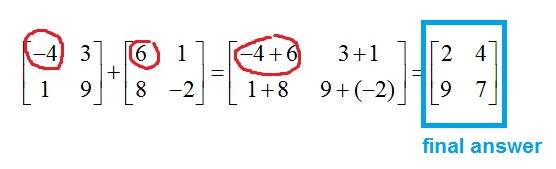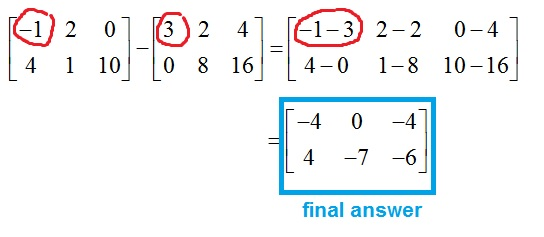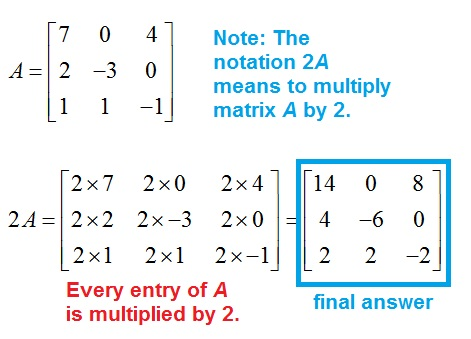﻿ Adding and subtracting matrices, and multiplying a matrix by a constant - MathBootCamps

# Adding and subtracting matrices, and multiplying a matrix by a constant

When multiplying a matrix by a scalar (a constant or number), or adding and subtracting matrices, the operations are done entry by entry. Let’s look at each operation separately to see how that works.

To add two matrices, add corresponding entries, as shown below. Notice that you need the matrices to be the same size in order for this to make sense.If the matrices are different sizes, the addition is undefined.## Subtracting matrices

Subtracting matrices works in the same way. You can subtract entry by entry.Just as with addition, this would be undefined if the matrices were different sizes. In that situation, your answer would simply be “undefined”.

## Multiplying a matrix by a constant (scalar multiplication)

The multiplication of a matrix by a constant or number (sometimes called a scalar) is always defined, regardless of the size of the matrix. You just need to make sure that each entry in the matrix is multiplied by the number.## Combining operations

In some questions, you might be asked to combine addition, subtraction, and multiplication by a constant. Here, we will look at a couple of examples to make sure you know how to approach these.

### Example

Find $$-2A + B$$ for:

$$A= \left[\begin{array}{cc} -4 & 1\\ 2 & -2\\ \end{array}\right]$$ and $$B= \left[\begin{array}{cc} 9 & -4\\ 0 & 8\\ \end{array}\right]$$

Remember to multiply each entry by the constant and to work entry by entry when adding.

\begin{align} -2A + B &= 2\left[\begin{array}{cc} -4 & 1\\ 2 & -2\\ \end{array}\right] + \left[\begin{array}{cc} 9 & -4\\ 0 & 8\\ \end{array}\right]\\ &= \left[\begin{array}{cc} -2 \times -4 & -2 \times 1\\ -2 \times 2 & -2 \times -2\\ \end{array}\right] + \left[\begin{array}{cc} 9 & -4\\ 0 & 8\\ \end{array}\right]\\ &= \left[\begin{array}{cc} 8 & -2\\ -4 & 4\\ \end{array}\right] + \left[\begin{array}{cc} 9 & -4\\ 0 & 8\\ \end{array}\right]\\ &= \left[\begin{array}{cc} 8 + 9 & -2 + (-4) \\ -4 + 0 & 4 + 8\\ \end{array}\right]\\ &= \boxed{\left[\begin{array}{cc} 17 & -6 \\ -4 & 12\\ \end{array}\right]}\end{align}

This also works when you have more than two matrices, as seen in the next example.

### Example

Find $$A – 3B + 2C$$ for:

$$A= \left[\begin{array}{cc} 1 & 1\\ 0 & 0\\ \end{array}\right]$$ , $$B= \left[\begin{array}{cc} 2 & 1\\ 1 & 4\\ \end{array}\right]$$, and $$C= \left[\begin{array}{cc} 5 & 2\\ 3 & 0\\ \end{array}\right]$$

\begin{align} A – 3B + 2C &= \left[\begin{array}{cc} 1 & 1\\ 0 & 0\\ \end{array}\right] – 3\left[\begin{array}{cc} 2 & 1\\ 1 & 4\\ \end{array}\right] + 2\left[\begin{array}{cc} 5 & 2\\ 3 & 0\\ \end{array}\right]\\ &= \left[\begin{array}{cc} 1 & 1\\ 0 & 0\\ \end{array}\right] – \left[\begin{array}{cc} 3\times2 & 3\times1\\ 3\times1 & 3\times4\\ \end{array}\right] + \left[\begin{array}{cc} 2\times5 & 2\times2\\ 2\times3 & 2\times0\\ \end{array}\right]\\ &= \left[\begin{array}{cc} 1 & 1\\ 0 & 0\\ \end{array}\right] – \left[\begin{array}{cc} 6 & 3\\ 3 & 12\\ \end{array}\right] + \left[\begin{array}{cc} 10 & 4\\ 6 & 0\\ \end{array}\right]\\ &= \left[\begin{array}{cc} 1 – 6 + 10 & 1 – 3 + 4\\ 0 – 3 + 6 & 0 – 12 + 0\\ \end{array}\right]\\ &= \boxed{\left[\begin{array}{cc} 5 & 2\\ 3 & – 12\\ \end{array}\right]}\end{align}

## Summary

Remember the following for operations on matrices:

• To add or subtract, go entry by entry.
• Addition and subtraction are only defined if the matrices are the same size.
• Scalar multiplication is always defined – just multiply every entry of the matrix by the scalar.

## Continue your study of matrix operations

Next: Multiplying matrices## Subscribe to our Newsletter!

We are always posting new free lessons and adding more study guides, calculator guides, and problem packs.

Sign up to get occasional emails (once every couple or three weeks) letting you know what's new!